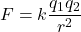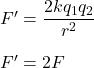Question

What happens to the force between two charges if one of the charges are doubled?
it decreased by a quarter
it doubles
it cuts in half

1.Euphemia

The new force between the charges becomes double of the initial force.

Explanation:

The force acting between charge particles is given by :k is electrostatic constant

r is distance between charges

If one of the charges are doubled, then, q₁ = 2q₁

The new force becomes,So, the new force between the charges becomes double of the initial force.